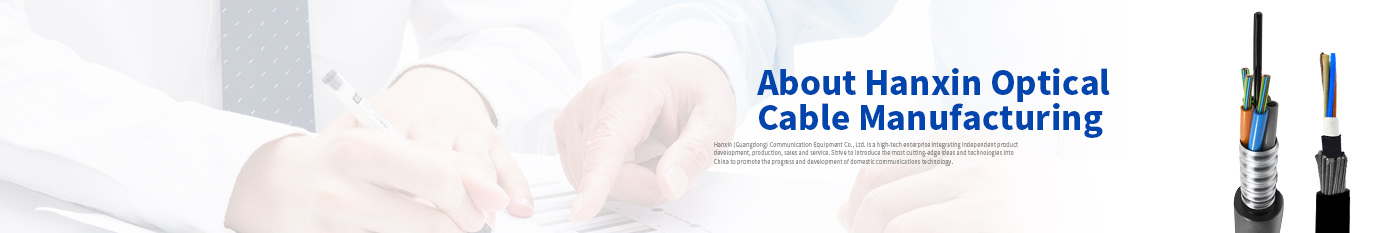Hanxin (guangdong) Communication Equipment Co., Ltd.## News

For the various types of loss there are various fiber loss formulae that can be used, they are as follows;

• The Total Link Loss = Cable Attenuation + Connector Loss + Splice Loss
• Cable Attenuation (dB) = Maximum Cable Attenuation Coefficient (dB/km) × Length (km)
• Connector Loss (dB) = Number of Connector Pairs × Connector Loss Allowance (dB)
• Splice Loss (dB) = Number of Splices × Splice Loss Allowance (dB)

These formulae show that the total loss is the sum of the worst variables within the various fiber segments. (Please note that the loss calculated in this way is an estimation as there various factors that influence the results.)